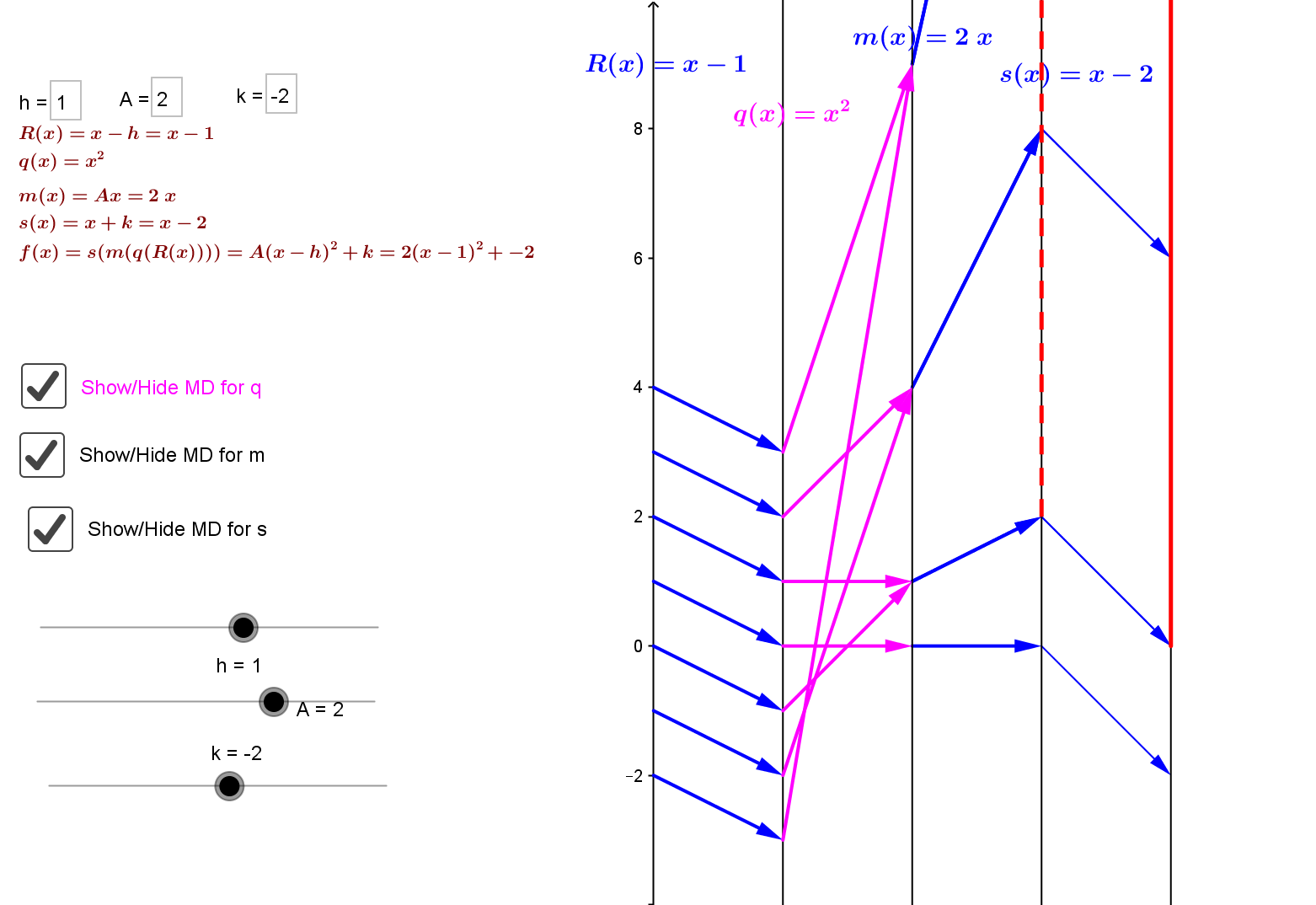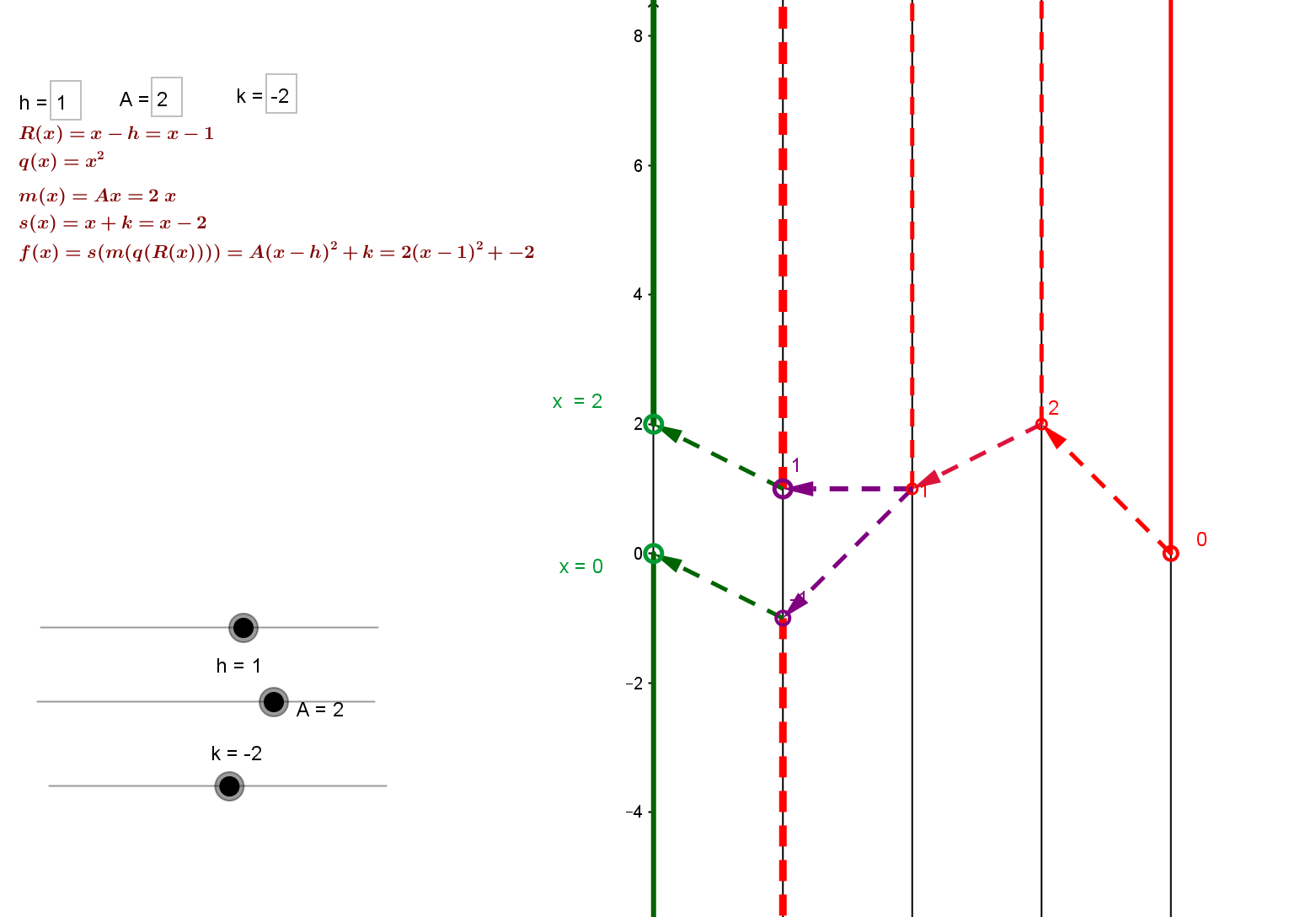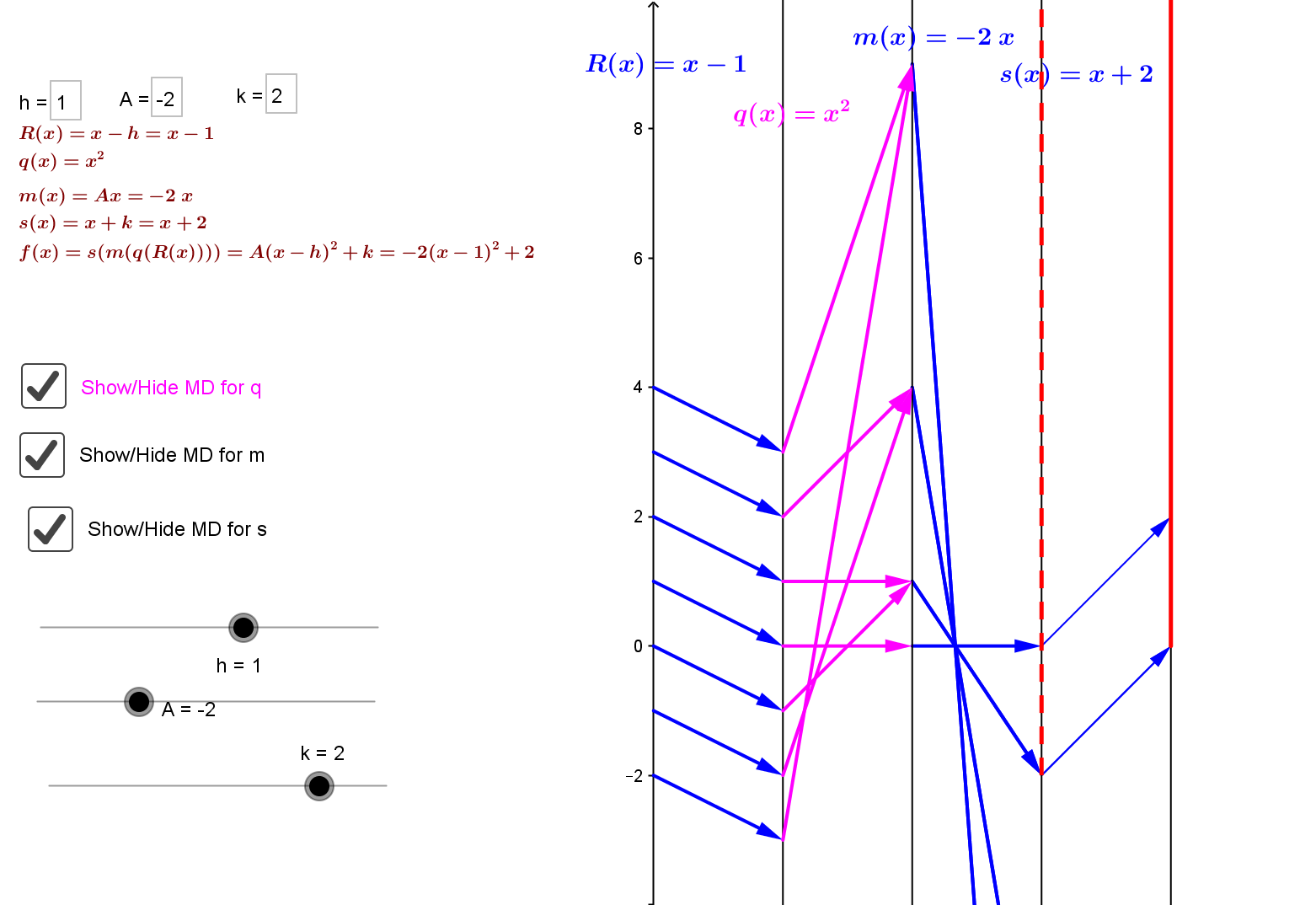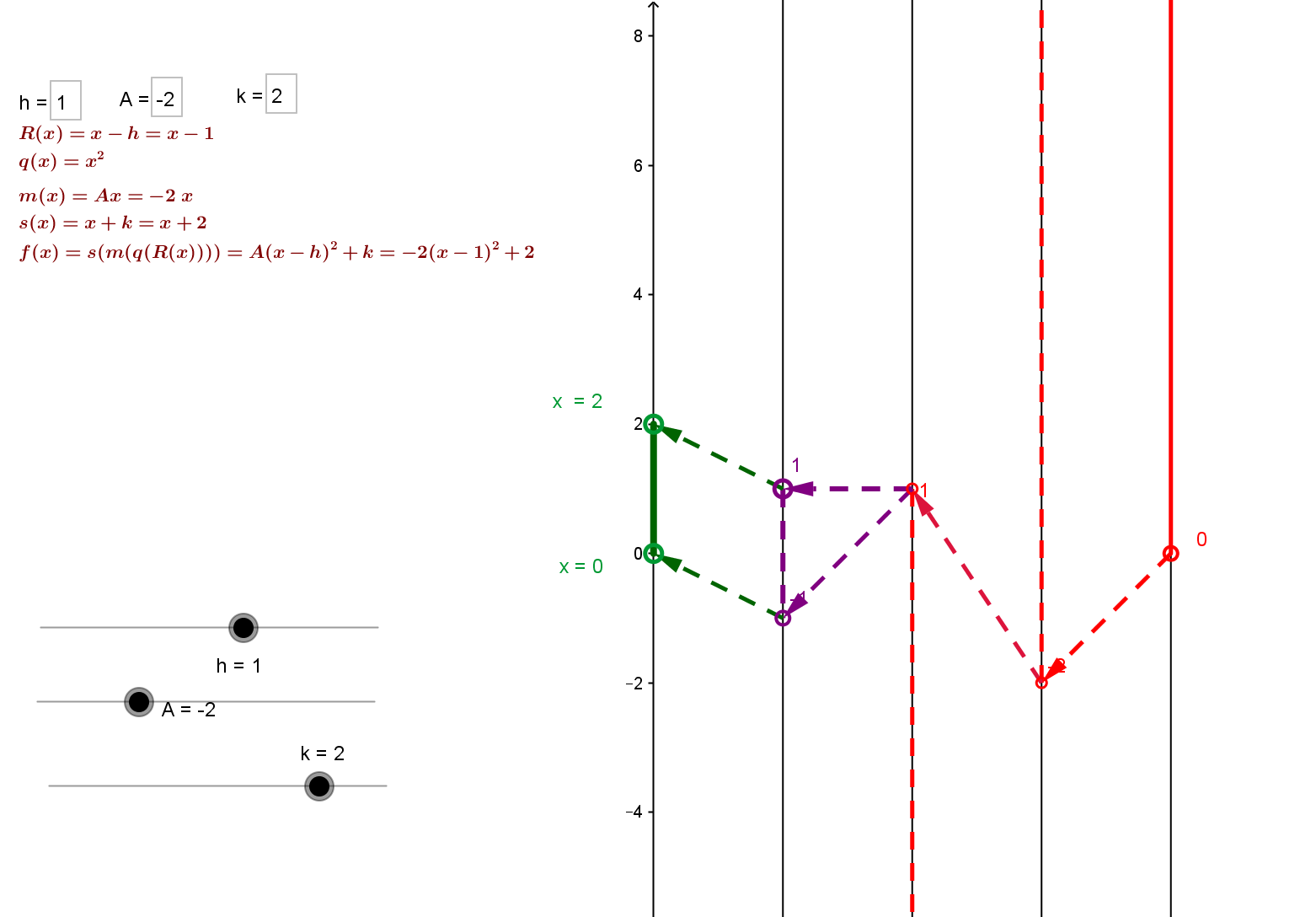Consider the quadratic function $f(x) = Ax^2 + Bx + C$.
The key result on quadratic functions as compositions is very useful here for visualizing how to solve an inequality of the form $f(x)=Ax^2 + Bx +C > 0$ with $A \ne 0$ by considering the related inequality $A(x−h)^2+k > 0$.

The mapping diagram for $f$ considered as a composition helps visualize the inequality $A(x−h)^2+k > 0$. as well as the solution set:
when $A>0, k \le 0$ the mapping diagram has solution set  $(-\infty, h - \sqrt{-\frac kA}) \cup (h + \sqrt{-\frac kA},\infty)$,
e.g., $2(x−1)^2-2 > 0$, the solution set is $(-\infty, 0)) \cup (2,\infty)$.while when $A<0, k>0$ the mapping diagram helps visualize the solution set, $(h - \sqrt{-\frac kA}) , h + \sqrt{-\frac kA})$ ,
e.g., $-2(x−1)^2+2 > 0$, the solution set is $(0 , 2)$..In the case, $A>0, k> 0$, the diagram visualizes  why the solution set is $(-\infty, ,\infty)$; when $A<0, k\le 0$ the diagram visualizes why the solution set is empty.

Example QF.QInEQ.0 Suppose $f(x)=2(x-1)^2 - 8 > 0$. Find the solution set, i.e., $\{x:f(x)>0\}$.
You can use this next dynamic example to solve quadratic equations like that in Example QF.QInEQ.0 visually with a mapping diagram of $f$.

Example QF.DQInEQ.0 Dynamic views for solving an equation $f(x) = A(x−h)^2+k > 0$ with mapping diagrams.